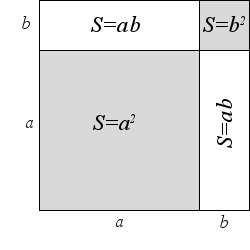Study of mathematics online.
Study math with us and make sure that "Mathematics is easy!"

# Square of the sum

Definition.
The square of the sum of two expressions is equal to the square of the first, plus twice the product of the first and second, plus the square of the second:

(a + b)2 = a2 + 2ab + b2

## Derivation of the formula of square of the sum

The proof of the formula is very simple. To prove the formula is sufficient to multiply the expression:

(a + b)2 = (a + b)·(a + b) = a2 + ab + ba + b2 = a2 + 2ab + b2

## Applying of square of the sum formula

Square of the sum formula convenient to use:
• to disclose the brackets
• to simplify expressions
• to calculate the squares of large numbers without using a calculator or multiplication in column

## Geometric illustrationFormula of square of the sum of two positive integers a and b can be illustrate a geometrically.

Consider a square with side (a + b), its area is (a + b)2.

Subdivided this square into four parts by straight lines.

Two of the parts are gray squares with the side sizes a and b. Areas of two smaller squares are a2 and b2 respectively.

Two other parts are congruent white rectangles with the side sizes a and b each. The area of each of the two rectangles is ab

Then area of the large square is the sum of the areas of its parts, the Figure illustrates the square of the sum formula:

(a + b)2 = a2 + b2 + ab+ ab = a2 + 2ab + b2

Example 1.
Expand brackets (x + 3)2.

Solution: Apply the square of the sum formula.

(x + 3)2 = x2 + 2·3·x + 32 = x2 + 6x + 9
Example 2.
Expand brackets (2x + 3y2)2.

Solution: Apply the square of the sum formula.

(2x + 3y2)2 = (2x)2 + 2·(2x)·(3y2) + (3y2)2 = 4x2 + 12xy2 + 9y4
Example 3.
Simplify the expression 9x2 + 6x + 1(3x + 1).

Solution: Apply the square of the sum formula in numerator.

9x2 + 6x + 1(3x + 1) = (3x + 1)2(3x + 1) = 3x + 1
Note that using square of the sum formula is easily find the squares of large numbers without using a calculator or multiplication in a column.
Example 4.
Apply the square of the sum formula to calculate 712.

Solution:

712 = (70 + 1)2 = 702 + 2·70·1 + 12 = 4900 + 140 + 1 = 5041